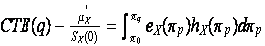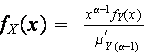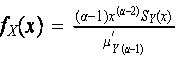Math 5637 (395) Risk Theory

Fall 2008

Instructor – James G. Bridgeman

syllabus for the course

(note use of the EXCEL functions OFFSET and SUMPRODUCT)

Distribution fitting example (pp144-45)

EXCEL Example for Panjer Recursion (try convolution on this one first!)

Example of Compound Geometric and Panjer Recursion For Ruin Probabilities

Not required at all, but if you wanted additional background and context you could read Sec. 6.8-6.10 and Sec. 8.5.

Cumulative Assignments (Final)

Study the Two Ruin Theory Notes above and the spreadsheet example for ruin probabilities

Sec. 8.1-8.4 and exerc. 8.1-8.3, 8.6-8.7, 8.9-8.18

Sec. 7.1-7.2

Study the Stop-Loss Example and Spreadsheet above … be able to do such problems independently

Study the EXCEL examples and distribution fitting examples above and be able to do such calculations independently.

Exerc. 6.26-6.27, 6.29-6.35, 6.37-6.58

Sec. 6.4-6.7

For the example on pages 143 to 145 (same as convolution example above) find each of the 5 two parameter distributions (normal, lognormal, inverse Gaussian, gamma, Pareto) that best fits the mean and variance of the aggregate losses.  Which of the 5 is the best choice? Hand in this work on the 17th

Sec. 6.1-6.3 and exer. 6.1-6.25

Sec. 4.6.10-4.6.11, 5.6 and exer. 4.56-4.60, 4.62,5.24-5.27

Sec. 4.6.7-4.6.9 and Exer. 4.41-4.47, 4.50-4.55

Calculate the coefficients of variation, skewness and kurtosis for the Poisson, Neg. Binomial, and Binomial distributions

Sec. 4.6.1-4.6.6 and exer. 4.40, 4.42-4.49, and use Faa’s formula to calculate the first 4 raw and central moments of the Poisson, Neg. Binomial, and Binomial distributions

Validate (comparing formulas is good enough, but surface interpretation is interesting so you might want to try it) that if X is a log-logistic then the k-th conditional tail moment distribution of X is a transformed beta (or, when γ=1, a generalized Pareto)

Determine the coefficient of skewness in example 5.15 (surface interpretation!)

Write down the formula for the 3rd moment analogous to Theorem 5.14 (surface interpretation!)

Sec. 5.1 – 5.5 and exer. 5.1 to 5.23 (in ch. 5 try to think in terms of the surface interpretation)

Read the Maximum Entropy Paper for background

Sec. 4.5 and exer. 4.37-4.39

Exer. 4.33-4.36

Sec. 4.3-4.4 and exer. 4.13-4.32 (keep a bookmark in appendix A!)

Sec. 4.1-4.2 and exer. 4.1-4.5, 4.7-4.9, 4.11-4.12

Sec. 3.3 and exer. 3.20-3.24

Sec. 3.1-3.2 and Exer. 3.1-3.19

Ch. 2 and Exer.2.1-2.5

Project Topics: (pick any six to submit by end of semester … topics will be added as we go)

#1 Critique the “proof” given in class that vanishing of y kS(y) as y goes to infinity implies existence of the k-th moment (assume non-negative support and nice behavior for y near 0)

#2 The surface interpretation shows that the size of a stationary population is proportional to the average lifetime (expectation of life at birth).  What does the surface interpretation say about the size of a stably growing population (the rate of births at time t is Bt = B0ek t for some constant k)?

#3 Use the surface interpretation and a little bit of algebra to write formulas for E[Xj(X^d)k] (or combinations thereof) for as many combinations of j=1,2,3,4 and k=1,2,3,4 as you can.

#4 Develop a purely algebraic method to express E[(X-d)+k] in terms of E[Xj] and E[(X^d)j] for j < k.  Show how it works for k = 1, 2, 3, 4.  Do not use the surface interpretation (as I do in class) and do not use integration by parts (as the textbook does) in any way, but use only algebra.

#5 Make three dimensional visual illustrations for the surface interpretation, including 2nd and 3rd moments and the relation of e(d), e2(d), and e3(d) to E[X],

E[X2], E[X3], E[X^d], E[(X^d)2], and E[(X^d)3].

#6 See handout on LX(u)=E[x^u].  Answer the series of questions.  (Think of a stationary population).

#7 Make a three dimensional visual illustration for the relationship below, and write down an interpretation in words.#8 Prove Faa’s Formula.

#9 Prove the Euler-Lagrange Differential Equation (rigorously) and explain in words and/or pictures why it is believable.

#10 Attach your name to our “no name” random variable by finding the probability density function for the distribution with maximum entropy on -∞ to ∞ subject to the constraints (I) it is a probability density (II) it has mean µ (III) it has dispersion function d(x)=ln{ln[e^((x-µ)/b)+e^(-(x-µ)/b]}, i.e. the integral of d(x)f(x) over -∞ to ∞ is one.

#11 Work out the definitions and properties of a family of severity distributions analogous to the transformed beta family, but based upon transformations of the log-Laplace distribution rather than the log-logistic.

#12 Work out the definitions and properties of a family of severity distributions analogous to the transformed beta family, but based upon transformations of the lognormal distribution rather than the log-logistic.

#13 Work out the definitions and properties of a family of severity distributions analogous to the transformed beta family, but based upon transformations of the no-name distribution (from #10) rather than the log-logistic.

#14 (speculative) Work out (by working backwards) what constraints in a maximum entropy derivation correspond to each member of the transformed gamma and transformed beta families.

#15 Work out what happens in the transformed beta and transformed gamma families if you replace the α-th conditional tail moment distributions:with the α-th equilibrium distributions):How do the resulting distributions differ from the gamma, transformed gamma, generalized Pareto, and transformed beta (that arose from the α-th conditional tail moment distributions)?

#16 Work out the definitions and properties of a true inverse logistic, inverse logistic and reciprocal inverse logistic family of distributions, analogous to the true inverse Gaussian, inverse Gaussian and reciprocal inverse Gaussian presented in class.

#17 Work out the definitions and properties of a family of severity distributions analogous to the transformed beta family, but based upon transformations of any one (you pick one) of the inverse logistic family of distributions developed in project #16, rather than the log-logistic.

#18 Work out the definitions and properties of a family of severity distributions analogous to the transformed beta family, but based upon transformations of the true inverse Gaussian distribution presented in class rather than the log-logistic.

#19 Prove (or, if a formal proof eludes you, just illustrate and discuss the connections) that the negative binomial is like a Poisson with contagion; i.e. the negative binomial with parameters (r,βt) gives the number of events in time t if the probability of one event in infinitesimal time t to t+dt, conditional on exactly m events having occurred from time 0 to time t, is equal to dt(rβ)((1+m/r)/(1+βt)).   Try to make a similar interpretation of the binomial distribution.

#20 Work out the parameter space for the the (a,b,2) family of frequency distributions; include an analysis of the distributions on the line r = -1, analogous to the geometric (r = 1) and logarithmic distributions (r = 0).  Does any kind of interesting series summation arise (analogous to the geometric and logarithmic series)?

#21 Show (using probability generating functions) that a mixed Poisson distribution with infinitely divisible mixing distribution is also a compound Poisson, and give two specific examples of the phenomenon.  Explain clearly why the infinite divisibility assumption is needed.

#22 (speculative) We have see that the Negative Binomial can be the result of a Poisson mixture or of a compound Poisson.  Can the Binomial distribution be the result of a Poisson mixture or of a compound Poisson?  If so give an example and work out the parameters.  If not, explain what goes wrong.

#23 Prove the Panjer recursion formula for an (a,b,1) primary distribution using Faa’s formula.

#24 Come up with a spreadsheet (or other programming) algorithm to generate sets {j k} with ∑ (k=1, ∞) j k k = n, n=0,1, 2, … Is this efficient enough to warrant replacing Panjer recursion with direct use of Faa’s formula to calculate compound distribution probabilities for (a, b, 0) primary distributions?  Note that this would give you a calculation technique anytime the probability generating function of the primary distribution is known, whether or not there is a recursive feature to it.  Is this an improvement versus brute force convolution?

#25 State and prove a usable generalization of Faa’s Formula for the case of three nested functions.

#26 Develop recursive approximation formulae for E[(x-d)+^3] in terms of E[(x-(d-h))+^3], S, and lower moments of (x-d)+; one formula for the discrete case (stair-step F) and one formula for the continuous case.

#27 Try to copy the development of ruin theory for the compound Poisson process using instead a compound Negative Binomial.   Point out exactly what goes wrong.  (speculative) Can you suggest or follow a way to keep going?